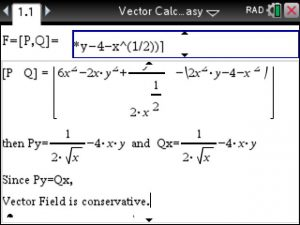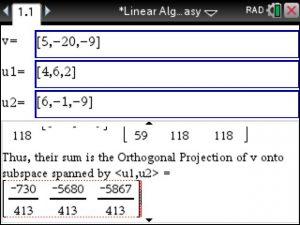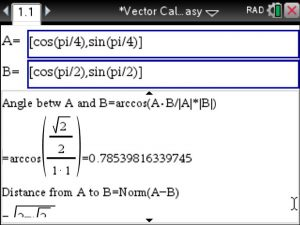## Tinspire CX CAS : Potential Function and Conservative Vector Field – Step by Step

To Find the Potential Function for a given Vector Field such as the one at http://tutorial.math.lamar.edu/Solutions/CalcIII/ConservativeVectorField/Prob4.aspx

use the Vector Calculus Made Easy app at https://www.tinspireapps.com/?a=VCME  , go to option 3,4

and enter the Vector Field as:Since the 2 partial derivatives match the Vector Field is conservative

and the Potential Function is found as shown below:Posted on Categories calculus, vector

## ▷Orthogonal Projection of v onto u1,u2 using the TiNSpire – Linear Algebra Made Easy

Say you need to find the orthogonal projection of v onto W the subspace of R^3  .

You pull out your TiNspire and launch the Linear Algebra Made Easy app from www.ti-nspire-cx.com and enter as follows:

.Now, just lean back and view the stepsuntil the final answer shows. Sweet!Posted on Categories linear algebra, matrix, vector

## Find the angle (Theta) between the two vectors using Vector Calculus Made Easy for the Ti-Nspire CX CAS

Say we have to..

Find the angle (Theta) between the two vectors

U= cos(pi/4)i + sin(pi/4)j

V= cos(pi/2)i + sin(pi/2)j

When entering these 2 vectors using option 1 6 in Vector Calculus Made Easy we will not use the i-j notation and instead use vector /matrix notation as  shown in this image . The angle is derived using arccos(AB/|A|*|B|) and given in radian format.Note: We know that the vectors differ by an angle of pi/2-pi/4=pi/4 or .785  , which also equals 45 degrees.

Posted on Categories angle, calculus, vector

## Video : Ableitungen, Stammfunktionen, Kurvendiskussion mit dem TiNspire CAS CX Programm

Video:  Schritt fuer Schritt  Analysis Leicht Gemacht auf dem TiNspire

-Spielerisch und ganz schnell lernt man Kurvendiskussion, Ableitungen, etc mit allen Schritten

-Ganz einfach zu benutzen und zu installieren!

-Laeuft nur auf dem TiNspire CAS CX

## Video: Vector Calculus & Analytic Geometry on the TI-Nspire CX CAS …

Watch the app in action!

More Details: http://www.tinspireapps.com/?a=VCME

Posted on Categories calculus, geometry, vector

## Step by Step Vector Calculus app now available for the TI-Nspire CX CAS …

TI-Nspire users can now do STEP BY STEP:

Vectors: Dot Product, Cross Product, Projection, Distance, ..

Vector Derivatives: Curl, Divergence, Laplacian, Curvature, ..

Line Integrals, Greens Theorem, Surface Integrals, Stokes Theorem

Analytic Geometry: Points, Lines, Planes

Multivariable Calculus: Tangent planes, Gradients, Lagrange Method, Laplace Transform,..

Linear Algebra: Row Echolon, Eigenvalues/vectors , Gram Schmidt Procedure, ..

Motion and Vector Values Functions

Parametric Equations

Conversions

and much more

More Details: http://www.tinspireapps.com/?a=VCME

Free trials.

Posted on Categories calculus, vector